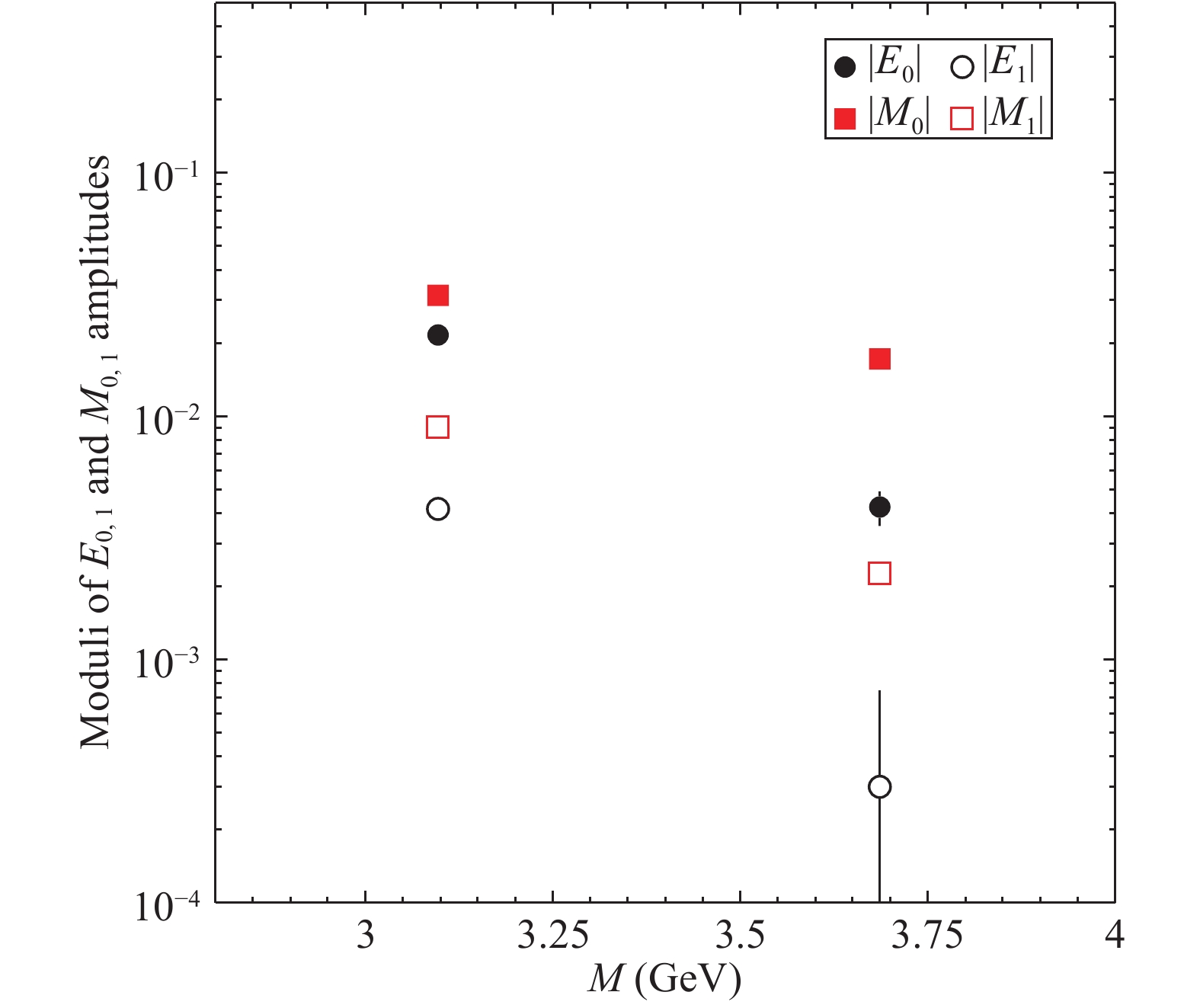# A model to explain the angular distribution of ${{{J /\psi}}}$and ${{\psi(2S)}}$decay into ${\Lambda \overline{\Lambda}}$and ${\Sigma^0 \overline{\Sigma^0}}$• BESIII data show a particular angular distribution for the decay of $J/\psi$and $\psi(2S)$mesons into $\Lambda \overline \Lambda$and $\Sigma^0 {\overline \Sigma}{}^0$hyperons: the angular distribution of the decay ${{\psi(2S)}} \to {\Sigma^0\overline{\Sigma}{}^0}$exhibits an opposite trend with respect to the other three channels: $J/\psi \to \Lambda \overline \Lambda$, $J/\psi \to \Sigma^0 {\overline \Sigma}{}^0$and $\psi(2S) \to \Lambda \overline \Lambda$. We define a model to explain the origin of this phenomenon.
••Get Citation
M. Alekseev, A. Amoroso, R. Baldini Ferroli, I. Balossino, M. Bertani, D. Bettoni, F. Bianchi, J. Chai, G. Cibinetto, F. Cossio, F. De Mori, M. Destefanis, R. Farinelli, L. Fava, G. Felici, I. Garzia, M. Greco, L. Lavezzi, C. Leng, M. Maggiora, A. Mangoni, S. Marcello, G. Mezzadri, S. Pacetti, P. Patteri, A. Rivetti, M. Da Rocha Rolo, M. Savrié, S. Sosio, S. Spataro and L. Yan. A model to explain the angular distribution of ${{{J /\psi}}}$and ${{\psi(2S)}}$decay into ${\Lambda \overline{\Lambda}}$and ${\Sigma^0 \overline{\Sigma^0}}$[J]. Chinese Physics C, 2019, 43(2): 1-1. doi: 10.1088/1674-1137/43/2/023103
M. Alekseev, A. Amoroso, R. Baldini Ferroli, I. Balossino, M. Bertani, D. Bettoni, F. Bianchi, J. Chai, G. Cibinetto, F. Cossio, F. De Mori, M. Destefanis, R. Farinelli, L. Fava, G. Felici, I. Garzia, M. Greco, L. Lavezzi, C. Leng, M. Maggiora, A. Mangoni, S. Marcello, G. Mezzadri, S. Pacetti, P. Patteri, A. Rivetti, M. Da Rocha Rolo, M. Savrié, S. Sosio, S. Spataro and L. Yan. A model to explain the angular distribution of ${{{J /\psi}}}$and ${{\psi(2S)}}$decay into ${\Lambda \overline{\Lambda}}$and ${\Sigma^0 \overline{\Sigma^0}}$[J]. Chinese Physics C, 2019, 43(2): 1-1.Milestone
Received: 2018-10-23
Article Metric

Article Views(269)
PDF Downloads(17)
Cited by(0)
Policy on re-use
To reuse of Open Access content published by CPC, for content published under the terms of the Creative Commons Attribution 3.0 license (“CC CY”), the users don’t need to request permission to copy, distribute and display the final published version of the article and to create derivative works, subject to appropriate attribution.
###### 通讯作者: 陈斌, bchen63@163.com
• 1.

沈阳化工大学材料科学与工程学院 沈阳 110142

Title:
Email:

## A model to explain the angular distribution of ${{{J /\psi}}}$and ${{\psi(2S)}}$decay into ${\Lambda \overline{\Lambda}}$and ${\Sigma^0 \overline{\Sigma^0}}$• 1. Università di Torino, I-10125, Torino, Italy
• 2. INFN Sezione di Torino, I-10125, Torino, Italy
• 3. INFN Laboratori Nazionali di Frascati, I-00044, Frascati, Italy
• 4. INFN Sezione di Ferrara, I-44122, Ferrara, Italy
• 5. Institute of High Energy Physics, Beijing 100049, People's Republic of China
• 6. Università di Ferrara, I-44122, Ferrara, Italy
• 7. Università del Piemonte Orientale, I-15121, Alessandria, Italy
• 8. Università di Perugia, I-06100, Perugia, Italy
• 9. INFN Sezione di Perugia, I-06100, Perugia, Italy

Abstract: BESIII data show a particular angular distribution for the decay of $J/\psi$and $\psi(2S)$mesons into $\Lambda \overline \Lambda$and $\Sigma^0 {\overline \Sigma}{}^0$hyperons: the angular distribution of the decay ${{\psi(2S)}} \to {\Sigma^0\overline{\Sigma}{}^0}$exhibits an opposite trend with respect to the other three channels: $J/\psi \to \Lambda \overline \Lambda$, $J/\psi \to \Sigma^0 {\overline \Sigma}{}^0$and $\psi(2S) \to \Lambda \overline \Lambda$. We define a model to explain the origin of this phenomenon.

### HTML1.   Introduction2.   Amplitudes and branching ratios3.   Effective model4.   Results5.   ConclusionsAppendix A: Production cross section
Reference (18)
PDF查看关注分享

Top

### 目录/DownLoad:  Full-Size Img  PowerPoint